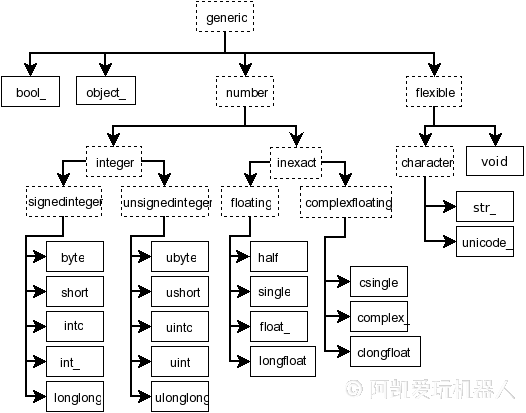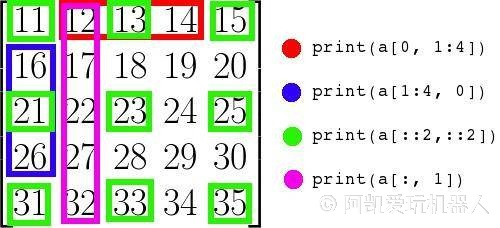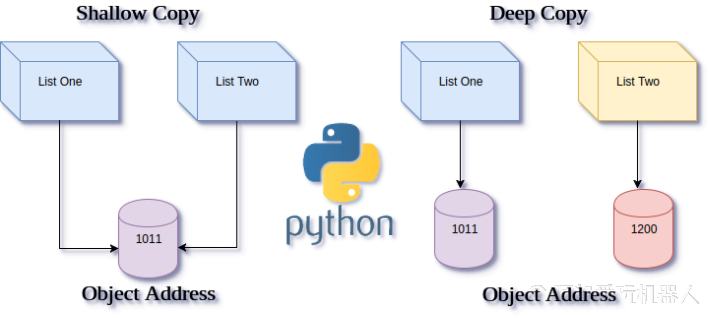# Numpy矩阵运算

## 1. Numpy简介NumPy是一个运行速度非常快的数学库，主要用于矩阵运算。NumPy是Python在科学计算领域取得成功的关键之一，如果你想通过Python学习数据科学，就必须学习NumPy。

• 矩阵运算功能

NumPy可以让你在Python中使用向量和数学矩阵，它封装了很多矩阵运算操作，这些都是list所不具备的。

• numpy更省空间

NumPy中数组的存储效率和输入输出性能均远远优于Python中等价的基本数据结构，且能够提升的性能是与数组中的元素成比例的。

• 处理速度更快

NumPy的大部分代码都是用C语言写的，其底层算法在设计时就有着优异的性能，这使得NumPy比纯Python代码高效得多。

## 2. 安装Numpy

pip install numpy


sudo pip3 install numpy


## 3. 引入Numpy模块

🖌 代码片段

import numpy


🖌 代码片段

import numpy as np


## 4. 设置打印选项

🖌 代码片段

# 设置Numpy的打印选项
# precision: 浮点数精确位数
# suppress:
# - True: 固定浮点 、
# - False: 使用科学计数法
np.set_printoptions(precision=3, suppress=True)


print(np.random.rand(10))


📓 输出日志

[0.469 0.484 0.284 0.089 0.156 0.431 0.479 0.737 0.825 0.921]


## 5. 数组初始化

NumPy的核心是多维数组 (ndarrays: n-dimension array)。

🖌 代码片段

# 一维数组
A1 = np.array([1, 2, 3])
print("A1 :\n{}".format(A1))


📓 输出日志

A1 :
[1 2 3]


🖌 代码片段

# 二维数组
A2 = np.array([[1, 2, 3], [4, 5, 6]])
print("A2 :\n{}".format(A2))


📓 输出日志

A2 :
[[1 2 3]
[4 5 6]]


## 6. 数组的属性

🖌 代码片段

print('A2.ndim = %d' % A2.ndim)
print('A2.shape')
print(A2.shape)
print('A2.size = %d' % A2.size)
print('A2.dtype = %s' % A2.dtype)
print('A2.itemsize = %d' % A2.itemsize)


📓 输出日志

A2.ndim = 2
A2.shape
(2, 3)
A2.size = 6
A2.dtype = int32
A2.itemsize = 4


## 7. 数组的数据类型  dtype 

 numpy  的ndarray所有的元素数据类型必须相同，这是为矩阵运算做保障的。

Python支持的数据类型有整型、浮点型、复数型，但这些类型不足以满足科学计算的需求，因此NumPy中添加了许多其他的数据类型，如：bool、inti、int64、float32、complex64等。同时，它也有许多其特有的属性和方法。🖌 代码片段

A =  np.array([[1, 2, 3], [4, 5, 6]])
print("转换前的格式: A.dtype = {}".format(A.dtype))
print("A:\n {}".format(A))
# 修改数据格式
A = A.astype("float32")
print('转换后 A.dtype = {}'.format(A.dtype))
print("A:\n {}".format(A))


📓 输出日志

转换前的格式: A.dtype = int32
A:
[[1 2 3]
[4 5 6]]

A:
[[1. 2. 3.]
[4. 5. 6.]]


## 8. 等差数列

### 8.1 整数等差数列

arange([start,] stop[, step,], dtype=None)

•  start  ：数值区间开始，默认是0
•  stop  ：数值区间结束
•  step  ：数值增加间隔，默认为1
•  dtype  ：数据类型

🖌 代码片段

print('递增数列: ')
print(np.arange(0, 10, 1))


📓 输出日志

递增数列:
[0 1 2 3 4 5 6 7 8 9]


🖌 代码片段

print(np.arange(10))


📓 输出日志

[0 1 2 3 4 5 6 7 8 9]


🖌 代码片段

print('递减数列')
print(np.arange(10, 0, -1))


📓 输出日志

递减数列
[10  9  8  7  6  5  4  3  2  1]


### 8.2 浮点数等差数列

🖌 代码片段

# 随机间隔
np.linspace(0, 10, 5)


📓 输出日志

array([ 0. ,  2.5,  5. ,  7.5, 10. ])


## 9. 数组变形🖌 代码片段

A = np.arange(10)


📓 输出日志

[0 1 2 3 4 5 6 7 8 9]


### 9.1 reshape

 reshape  函数变形前与变形后的 size 必须相同，否则就会报错。

 ndarray  对象  A  在调用  reshape  函数时, 返回一个新的ndarray对象，原来的ndarray并不发生改变。

reshape函数的原型：

reshape(a, newshape, order='C')

•  a  ：是要被resize的数组
•  new_shape  ：是新数组的尺寸，类型为tuple元组类型

🖌 代码片段

new_dim = (2,5) # 新的数组维度
B = np.reshape(A, new_dim)
print("B 尺寸: {}".format(B.shape))
print(B)
print("A 尺寸: {}".format(A.shape))
print(A)


📓 输出日志

B 尺寸: (2, 5)
[[0 1 2 3 4]
[5 6 7 8 9]]
A 尺寸: (10,)
[0 1 2 3 4 5 6 7 8 9]


🖌 代码片段

B = A.reshape(new_dim)


### 9.2 resize

np.resize的函数原型

resize(a, new_shape)

•  a  ：要被resize的数组
•  new_shape  ：新数组的尺寸，类型为tuple元组类型

🖌 代码片段

B = np.resize(A, new_dim)
print("B 尺寸: {}".format(B.shape))
print(B)
print("A 尺寸: {}".format(A.shape))
print(A)


📓 输出日志

B 尺寸: (2, 5)
[[0 1 2 3 4]
[5 6 7 8 9]]
A 尺寸: (10,)
[0 1 2 3 4 5 6 7 8 9]


 A.resize(new_dim)  函数会直接在原来的数组里面修改，不会返回新的数组。

🖌 代码片段

A.resize(new_dim)
print("A 尺寸: {}".format(A.shape))
print(A)


📓 输出日志

A 尺寸: (2, 5)
[[0 1 2 3 4]
[5 6 7 8 9]]


## 10. 线性代数运算  np.linalg 

numpy的  linalg  里包含着线性代数的函数。

🖌 代码片段

import numpy.linalg as LA


🖌 代码片段

A = np.random.rand(16).reshape((4, 4))


📓 输出日志

[[0.477 0.084 0.615 0.226]
[0.009 0.246 0.653 0.785]
[0.752 0.377 0.395 0.335]
[0.03  0.997 0.114 0.243]]


### 10.1 矩阵转置

🖌 代码片段

print(A.T)


📓 输出日志

[[0.477 0.009 0.752 0.03 ]
[0.084 0.246 0.377 0.997]
[0.615 0.653 0.395 0.114]
[0.226 0.785 0.335 0.243]]


### 10.2 矩阵求逆

🖌 代码片段

LA.inv(A)


📓 输出日志

[[-0.572 -0.414  1.717 -0.499]
[ 0.3   -0.344 -0.232  1.15 ]
[ 2.974 -0.207 -1.903  0.521]
[-2.563  1.559  1.637 -0.788]]


### 10.3 矩阵乘法

🖌 代码片段

# 创建矩阵B
B = np.random.rand(8).reshape((2, 4))
print("B = \n{}".format(B))


📓 输出日志

B =
[[0.401 0.394 0.528 0.917]
[0.513 0.735 0.289 0.533]]


🖌 代码片段

print(B.dot(A))


📓 输出日志

[[0.619 1.244 0.817 0.799]
[0.485 0.864 0.97  0.919]]


### 10.4 行列式求解

🖌 代码片段

print(LA.det(A))


📓 输出日志

0.1799090776573458


## 11. 随机数  np.random 

### 11.1 生成整数随机数组

🖌 代码片段

# 随机生成2x3的矩阵，矩阵元素取值范围 [low, high)
np.random.randint(low=0, high=100, size=(2,3))


📓 输出日志

array([[24, 13, 88],
[14, 22, 61]])


### 11.2 生成0-1区间的浮点数随机数组

🖌 代码片段

print('生成10个0-1区间内的随机数值')
print(np.random.rand(10))


📓 输出日志

生成10个0-1区间内的随机数值
[0.638 0.477 0.969 0.186 0.692 0.245 0.162 0.877 0.457 0.051]


### 11.3 生成满足正态分布的随机数组

🖌 代码片段

print('生成10个满足正态分布的随机数组')
print(np.random.randn(10))


📓 输出日志

生成10个满足正态分布的随机数组
[ 0.26  -0.702  0.107 -0.105 -2.571 -0.665  0.632 -2.242 -1.283  0.924]


### 11.4 打乱排序

🖌 代码片段

A = np.arange(10)
print("A = {}".format(A))
np.random.shuffle(A)
print("打乱顺序后\n A = {}".format(A))


📓 输出日志

A = [0 1 2 3 4 5 6 7 8 9]

A = [0 5 3 4 9 2 7 6 8 1]


### 11.5 随机抽样

🖌 代码片段

np.random.choice(A, size=2)


📓输出日志

array([7, 3])


## 12. 全局函数  ufunc 

•  +  加法
•  -  减法
•  *  乘法，注意这里不是点乘
•  /  除法
• 三角函数，数学函数

🖌 代码片段

# 随机生成2x3的矩阵，矩阵元素取值范围 [low, high)
A = np.random.randint(low=0, high=10, size=(3,3))
B = np.random.randint(low=0, high=10, size=(3,3))
print("A \n{} \nB\n{}".format(A, B))


📓 输出日志

A
[[7 9 5]
[5 3 0]
[1 5 9]]
B
[[5 1 8]
[4 6 6]
[1 4 6]]


### 12.1 全局加法

🖌 代码片段

A + B


📓 输出日志

array([[12, 10, 13],
[ 9,  9,  6],
[ 2,  9, 15]])


### 12.2 全局减法

🖌 代码片段

A - B


📓 输出日志

array([[ 2,  8, -3],
[ 1, -3, -6],
[ 0,  1,  3]])


### 12.3 全局乘法

🖌 代码片段

A * B


📓 输出日志

array([[35,  9, 40],
[20, 18,  0],
[ 1, 20, 54]])


### 12.4 全局除法

🖌 代码片段

A / B


📓 输出日志

array([[1.4  , 9.   , 0.625],
[1.25 , 0.5  , 0.   ],
[1.   , 1.25 , 1.5  ]])


### 12.5 其他全局函数

🖌 代码片段

np.sin(A)


📓 输出日志

array([[ 0.6569866 ,  0.41211849, -0.95892427],
[-0.95892427,  0.14112001,  0.        ],
[ 0.84147098, -0.95892427,  0.41211849]])


## 13. 索引操作  Index### 13.1 一维数组索引

Numpy中多维数组的索引操作与Python中list的索引操作一样，同样由  start  stop  step  三个部分组成。

🖌 代码片段

A = np.arange(10)


🖌 代码片段

A[:3]


📓 输出日志

array([0, 1, 2])


🖌 代码片段

A[0:3]


📓 输出日志

array([0, 1, 2])


### 13.2 多维数组索引## 14. 矩阵拼接

•  hstack()  横向拼接
•  vstack()  纵向拼接

🖌 代码片段

A = np.arange(16).reshape(4,4)
B = np.arange(16).reshape(4,4) * -1
print("A: \n{}".format(A))
print("B: \n{}".format(B))


📓 输出日志

A:
[[ 0  1  2  3]
[ 4  5  6  7]
[ 8  9 10 11]
[12 13 14 15]]
B:
[[  0  -1  -2  -3]
[ -4  -5  -6  -7]
[ -8  -9 -10 -11]
[-12 -13 -14 -15]]


🖌 代码片段

# 注意这里传入的是tuple
np.hstack((A, B))


📓 输出日志

array([[  0,   1,   2,   3,   0,  -1,  -2,  -3],
[  4,   5,   6,   7,  -4,  -5,  -6,  -7],
[  8,   9,  10,  11,  -8,  -9, -10, -11],
[ 12,  13,  14,  15, -12, -13, -14, -15]])


🖌 代码片段

np.vstack((A, B))


📓 输出日志

array([[  0,   1,   2,   3],
[  4,   5,   6,   7],
[  8,   9,  10,  11],
[ 12,  13,  14,  15],
[  0,  -1,  -2,  -3],
[ -4,  -5,  -6,  -7],
[ -8,  -9, -10, -11],
[-12, -13, -14, -15]])


## 15. 内存共享与深度拷贝

🖌 代码片段

A = np.array([[1, 2], [3, 4]])
B = A
B = -1
print("A =\n {}".format(A))
print("B =\n {}".format(B))


📓 输出日志

A =
[[-1  2]
[ 3  4]]
B =
[[-1  2]
[ 3  4]]


🖌 代码片段

A = np.array([[1, 2], [3, 4]])
B = np.copy(A)
B = -1
print("A =\n {}".format(A))
print("B =\n {}".format(B))


📓 输出日志

A =
[[1 2]
[3 4]]
B =
[[-1  2]
[ 3  4]]


🖌 代码片段

B = A.copy()